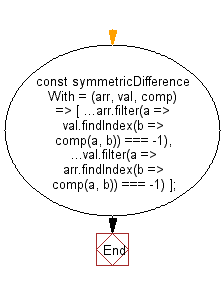# JavaScript: Get the symmetric difference between two given arrays, using a provided function as a comparator

## JavaScript fundamental (ES6 Syntax): Exercise-132 with Solution

Write a JavaScript program to get the symmetric difference between two given arrays, using a provided function as a comparator.

• Use Array.prototype.filter() and Array.prototype.findIndex() to find the appropriate values.

Sample Solution:

JavaScript Code:

``````//#Source https://bit.ly/2neWfJ2
const symmetricDifferenceWith = (arr, val, comp) => [
...arr.filter(a => val.findIndex(b => comp(a, b)) === -1),
...val.filter(a => arr.findIndex(b => comp(a, b)) === -1)
];

console.log(symmetricDifferenceWith(
[1, 1.2, 1.5, 3, 0],
[1.9, 3, 0, 3.9],
(a, b) => Math.round(a) === Math.round(b)
));
```
```

Sample Output:

```[1,1.2,3.9]
```

Flowchart:Live Demo:

See the Pen javascript-basic-exercise-132-1 by w3resource (@w3resource) on CodePen.

Improve this sample solution and post your code through Disqus

What is the difficulty level of this exercise?

Test your Programming skills with w3resource's quiz.

﻿